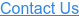# Fixed vs. Float Analysis

Forward Curve Accuracy – Hairy Graph

Many borrowers use forward curves to help project interest rates over the life of a project.  The following graph illustrates how accurately the market predicts LIBOR for the next five years at any given time.  Key takeaways:

• In general, the market substantially overestimates the path of LIBOR
• When the market underestimates the path of LIBOR, it is usually during a tightening cycle

The blue line is LIBOR.

The grey lines are forward curves, meaning what the market thought LIBOR would do for the next five years.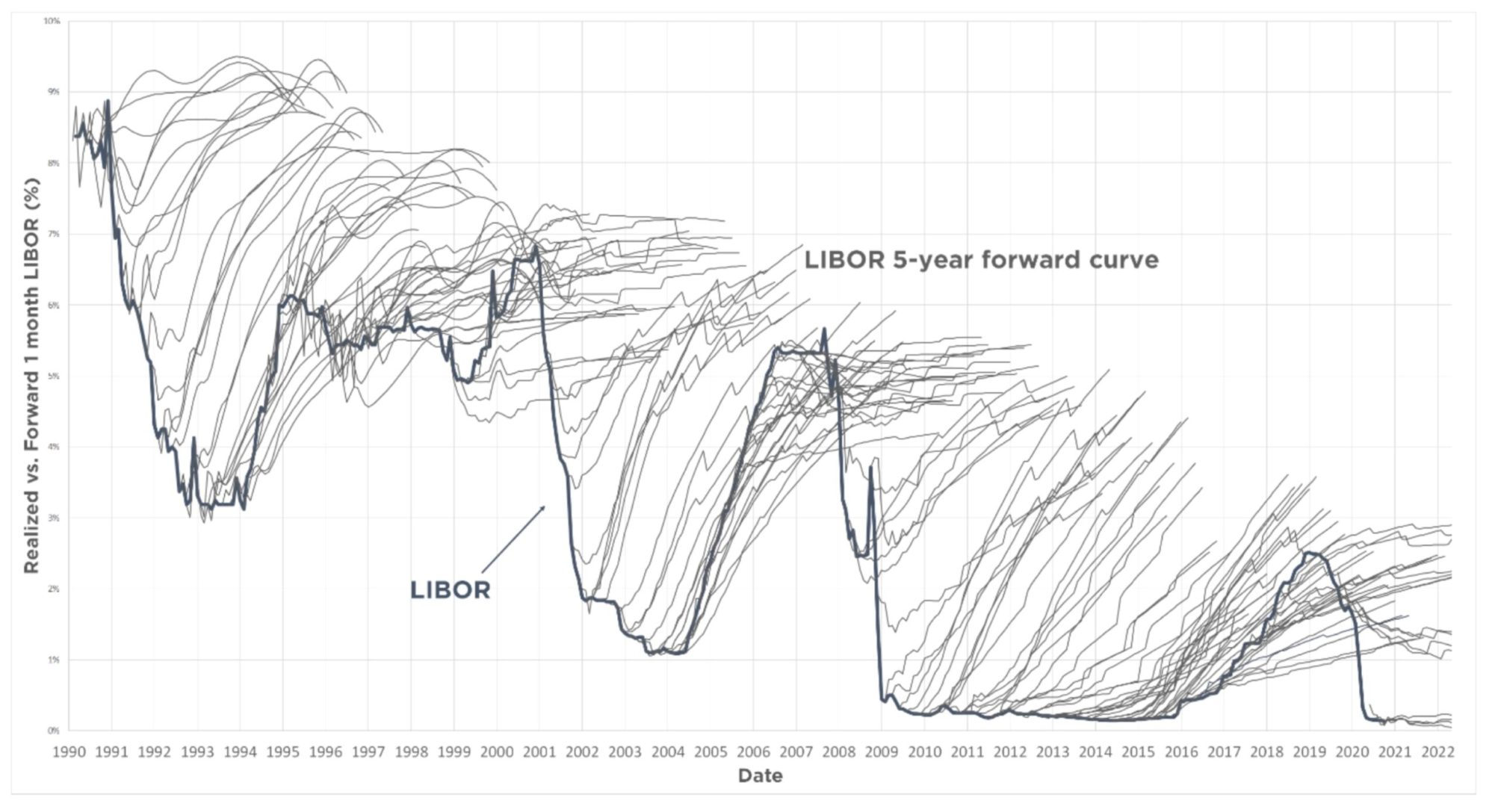Fixed vs Floating Cost – Quantifying the Difference

The following graphs quantify the historical difference between fixed and floating rates over a variety of tenors.

Swap vs What LIBOR Ends Up Doing

Red Line           – Swap Rate
Green Line         – this is the most important aspect of this graph.

• This line is what the floating rate averages over the next two years.
• In other words, if the green line is above the red line, you would have been better off locking in a fixed rate on that day.

Given the complexity of this graph, let’s select a data point and use it as an illustration.  Look at late-1998, when all three lines converge at roughly 5.70%.

Blue Line – LIBOR on 8/1/98 was 5.70%
Red Line – borrower could swap to fixed for two years at 5.70%
Green Line – if borrower stayed floating, the interest rate over the next two years (ending on 8/1/2000) would average 5.70%.

Conclusion – On 8/1/98, regardless of whether the borrower remained floating or swapped to fix, they paid 5.70% for the next two years.Now take a look at a different time horizon – 2007.

Blue Line – LIBOR on 10/1/07 was 5.23%.
Red Line – borrower could swap to fixed for two years at 4.66%.
Green Line – if borrower stayed floating, the interest rate over the next two years (ending on 10/1/09) would average 2.50%.

Conclusion – this borrower had a difficult decision to make on 10/1/07 because the fixed rate was actually lower than the floating rate.  If they remained floating, however, they ended up paying an average interest rate of just 2.50% because the Fed cut rates dramatically during the Great Recession.With the tutorial for this graph under our belt, let’s shift gears and take a look at another graph that illustrates the point above.

Cost/Savings of Fixed Rate

This graph helps to quantify the cost borrowers paid over the term by fixing the rate.  We used swap rates to represent the fixed rate.

When the cost is negative (the red bars in the graph below) a borrower would have been better off fixing the rate instead of staying floating.

This graph really aggregates the information from the graph above and more simply illustrates three things:

–  the cost/savings from fixing the rate
–  when was the best time to fix the rate
–  magnitude of cost/savings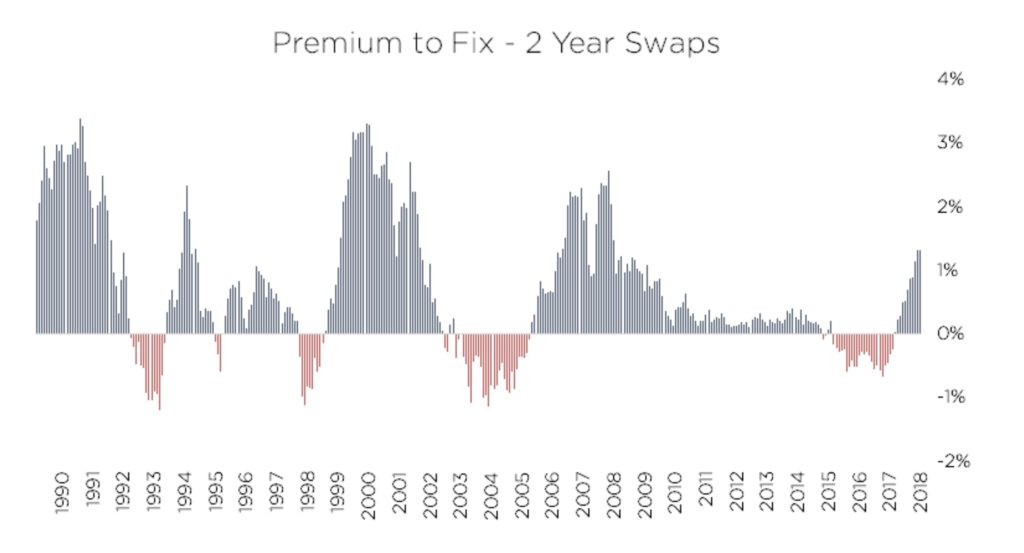With a better understanding of what these graphs illustrate, let’s look at how this analysis compares over different tenors beginning with the two-year graphs we just examined

2 Year Time Period – Better to Fix or Float?

• 6% of the time it was better to fix the rate
• the maximum savings by fixing the rate was 1.20% annually over the next two years
• the maximum savings by floating was 3.30% annually over the next two years5 Year Time Period – Better to Fix or Float?

• 8% of the time it was better to fix the rate
• the maximum savings by fixing the rate was 1.00% annually over the next five years
• the maximum savings by floating was 4.80% annually over the next five years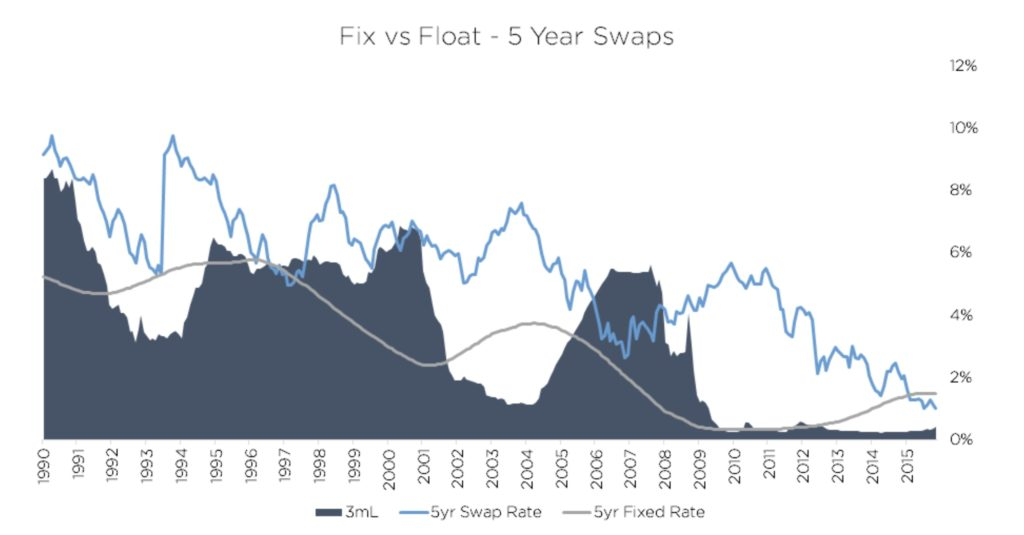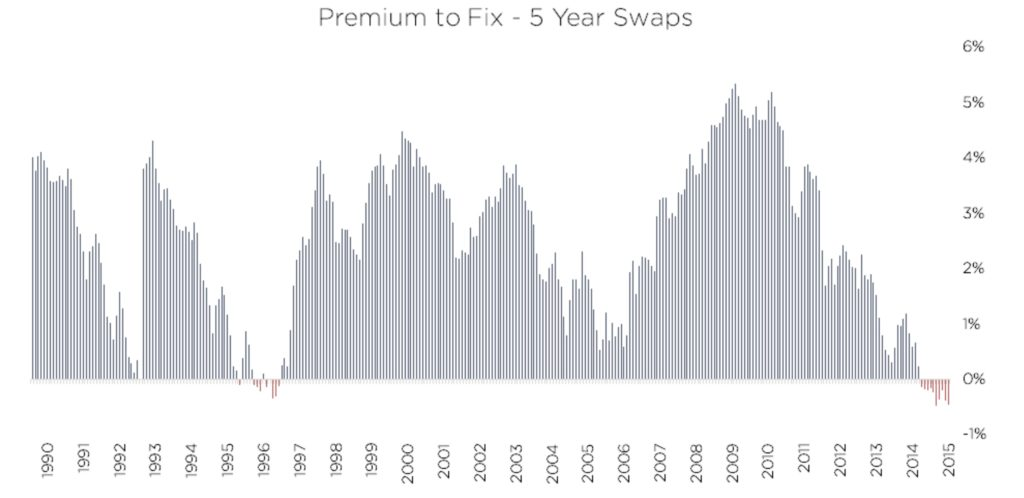10 Year Time Period – Better to Fix or Float?

• It has never been less expensive to fix the rate
• the maximum savings by floating was 4.70% annually over the next ten years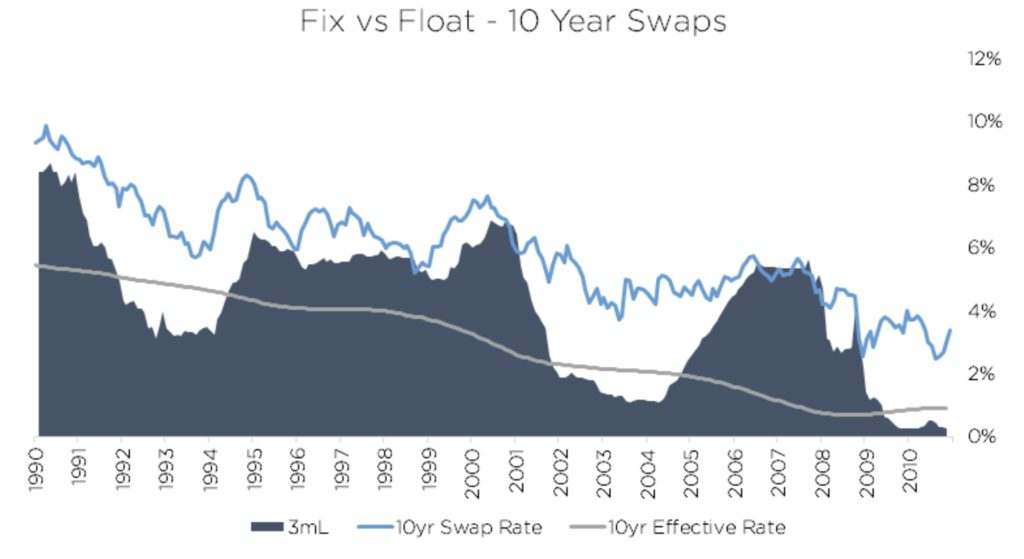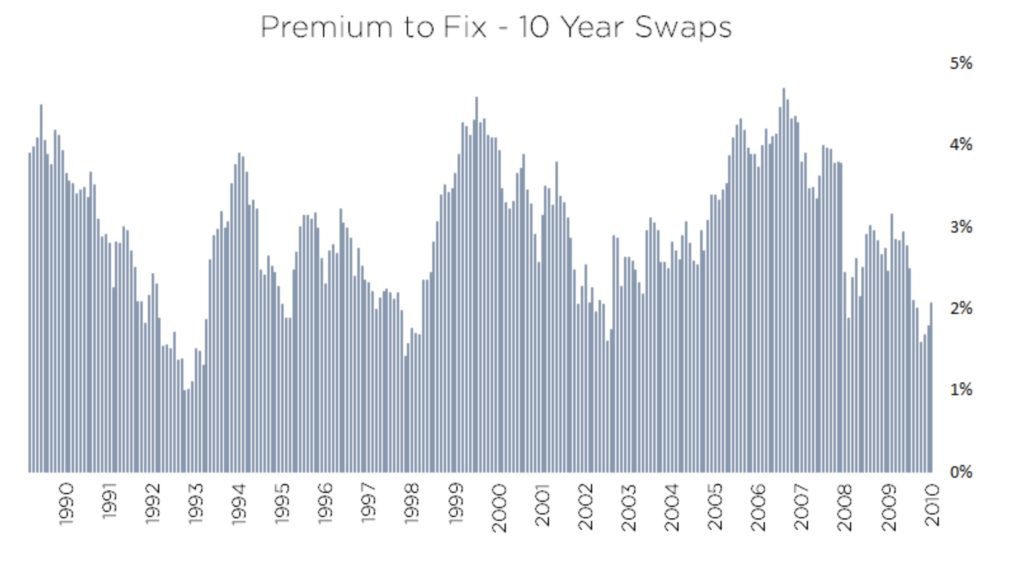Conclusions

Generally, the market dramatically overestimates the path of Fed Funds.  This explains why borrowers that remain floating generally save money vs the fixed rate alternative.

• The most notable exception to this, however, is during a tightening cycle. When the FOMC is hiking interest rates, the market tends to underestimate the path of Fed Funds.

While arguments could be made that this tightening cycle is different, this should be carefully weighed against the possibility of a dramatic shift in FOMC composition over the next year.

Asymmetric risk/reward – the magnitude of savings when floating is substantially greater than when fixed.

When it was right to be floating, it was right by a significant amount.

When it was wrong to be floating, it was wrong by a small amount.

The longer the term, the greater the likelihood of that floating interest rates will save money relative to the fixed rate alternative.